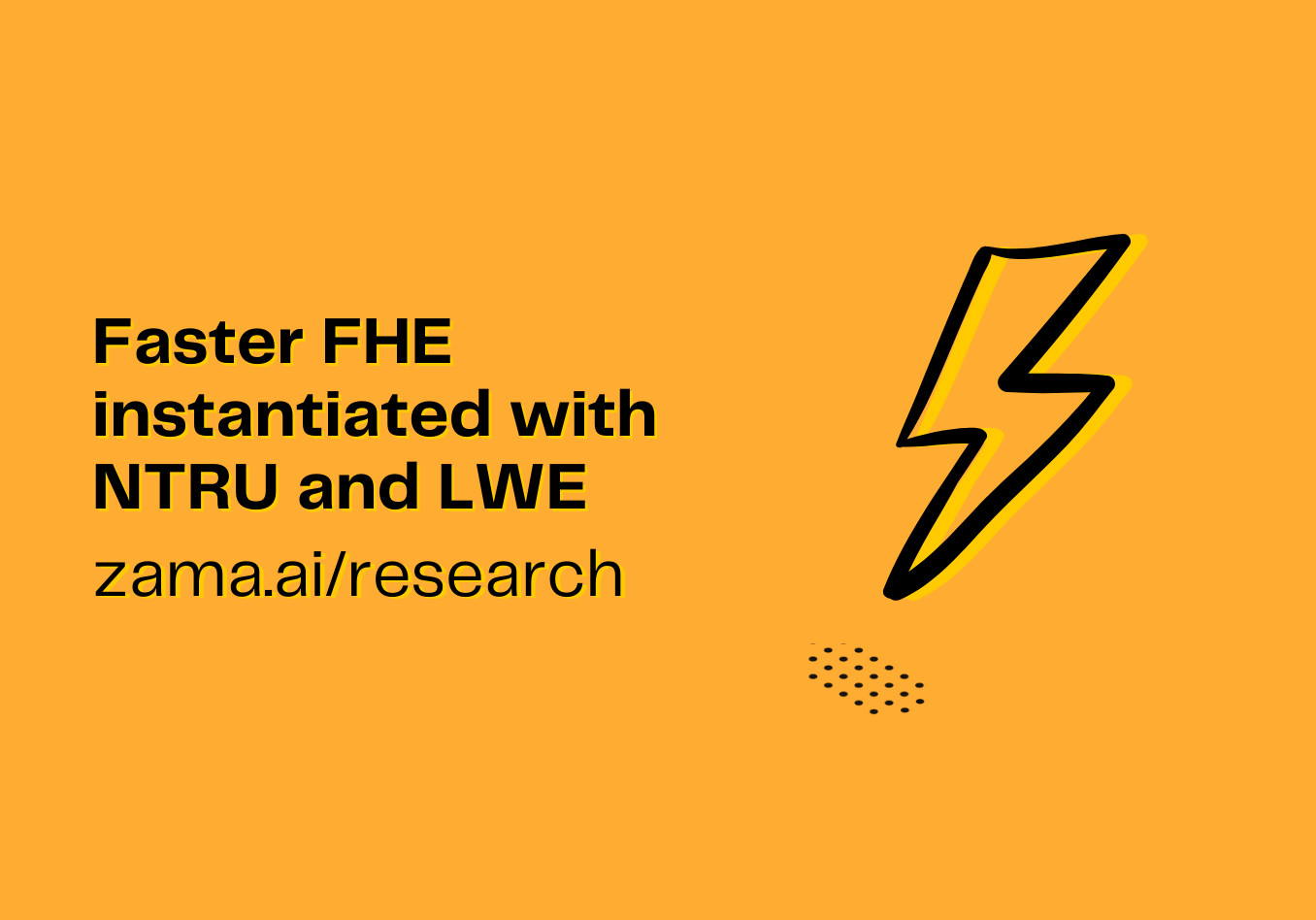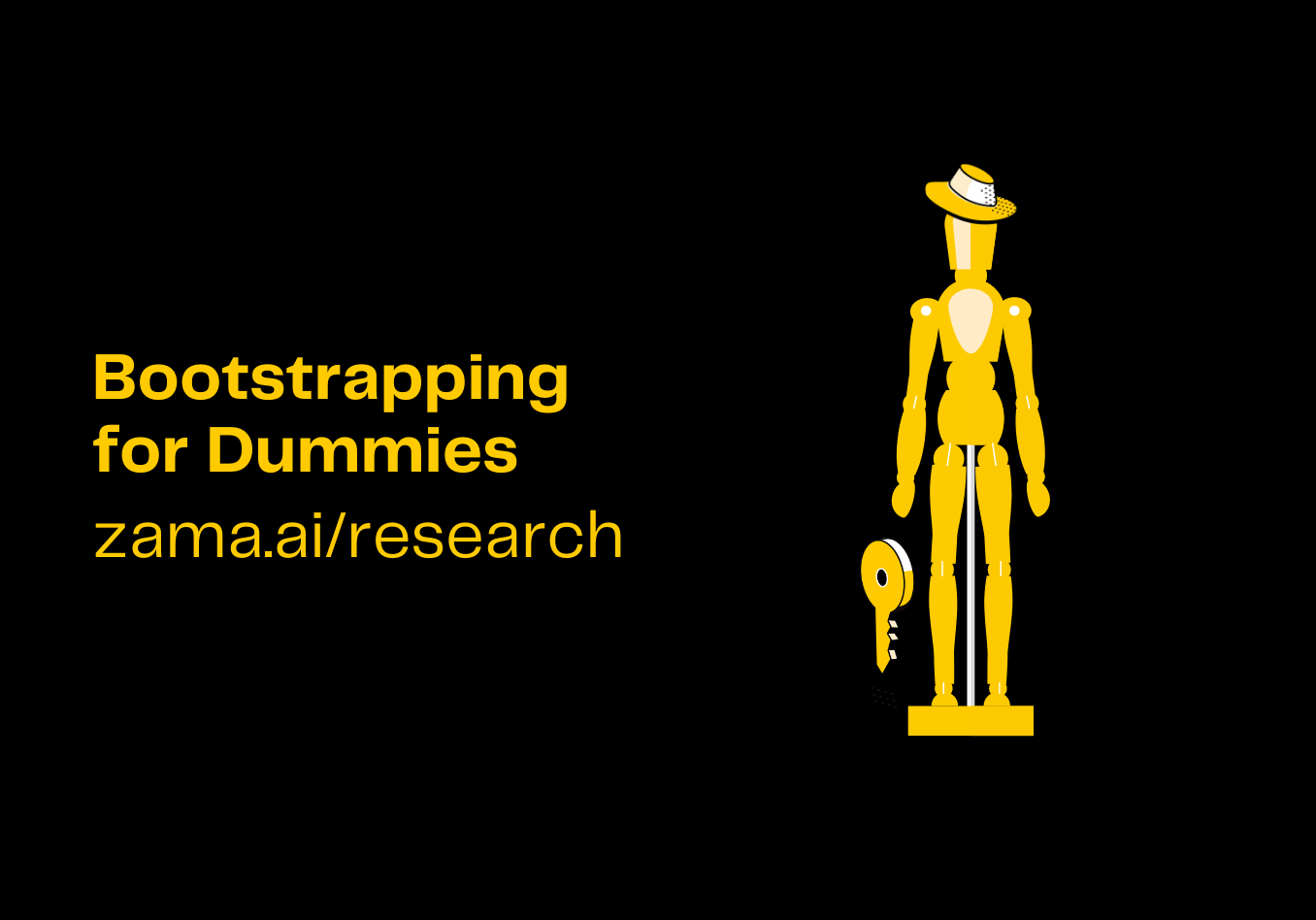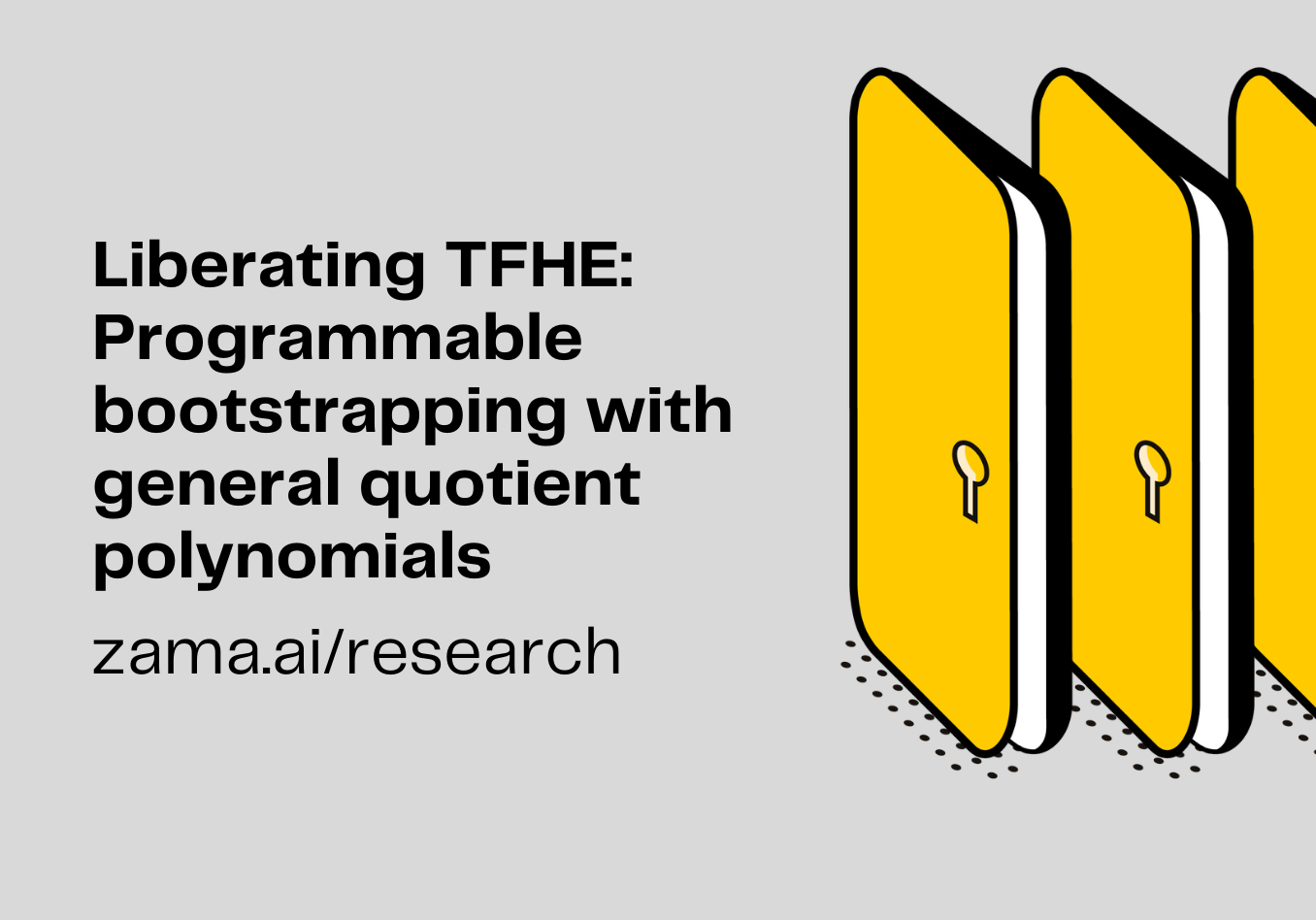When a student first learns cryptography, the normal route is to first look at the RSA encryption scheme. This is a really nice way into the subject, as it shows a simple scheme, based on elegant mathematics, which appears at first sight to be highly relevant to practice. However, this initial view turns out to be completely wrong. Indeed textbook RSA, namely encrypting by executing the function C = Me mod N is known to be insecure. Thus one is usually taught to use something like RSA-OAEP as an encryption scheme.

But even the RSA-OAEP encryption scheme is rarely used in practice. The ideas behind the RSA scheme are used in modern systems, but as just one piece in a complex jigsaw puzzle. Take, for example, the TLS protocol. Here, one usually encrypts actual messages using an AEAD symmetric cipher, whose key has been obtained by a complex interactive protocol. This protocol is based, in part, on the basic Diffie-Hellman protocol where one (or both) ends of the key exchange are authenticated using (possibly) an RSA-based digital signature, whose public key is contained in a certificate which has (normally) been signed by the RSA signature scheme. Thus we see that what looks like a simple, elegant mathematical construction is but one small piece of a complex jigsaw puzzle.

This is, in fact, a simple example of a protocol, in an encryption/secure messaging protocol, of which TLS is but one example. The adversary model is usually one of trying to protect the sender (Alice) and the receiver (Bob) from an adversary (Eve) who is sitting between them. Despite this simple example cryptographic service resulting in a complex combination of various cryptographic pieces, it is common (even for seasoned cryptographers) to think they can approach Fully Homomorphic Encryption (FHE) in the same way that the naive undergraduate approaches encryption via RSA. In this post we aim to explain why this is not the case, and how various other pieces need to be brought into play.

When we look at simple encryption, we see that we seem to have three entities: the sender, the receiver and the adversary. Indeed, this is a simplification. The reason the key agreement scheme in TLS is not a direct application of signed Diffie-Hellman is that TLS needs to cope simultaneously with various entities wanting to send messages to others and so forth. Thus we actually have many Alices, and many Bobs, and some of the Alices and Bobs could be controlled by Eves. What we require in TLS is that messages sent from an honest Alice to an honest Bob are delivered securely and authentically.

## The FHE Players

In FHE we have a similar, but even more complex situation: in it’s basic form we have four, not three, entities. An encryptor (let us call them Alice again), a decryptor (let us call them Bob again), someone doing the FHE computation (let us call them Charlie), as well as an adversary (which we will again call Eve). However, even in this simplified scenario in which we have a single Alice, a single Bob and a single Charlie, things are more complex.

Alice needs to trust Charlie to actually compute the function being required, otherwise information could leak to Bob. For example, suppose Alice encrypts a value x, and suppose that Charlie will compute the encryption of F(x) for some function F. Consider what would happen if Charlie replaces the function F with the identity function? In this case Bob would learn the value x. In this case we are assuming a collusion between Charlie and Bob. Actually, however, if Charlie and Bob are colluding then Charlie could actually decrypt the value x directly using Bob’s decryption key.

As another example consider what would happen if Charlie computes the function: If x<0 then output Enc(F(x)) Else Enc(garbage).

In this case if Charlie can observe whether Bob aborts what he does next, or signals an error, due to garbage being received (a so-called selective failure attack), then Charlie can determine information about x without needing to collaborate with Bob. Such selective failure attacks can actually be quite devastating, as Charlie can craft specific ciphertexts containing garbage which allow (after many executions of the protocol) Charlie to extract Bob’s secret key. These are like the original Bleichenbacher attacks on early versions of SSL.

So we need to protect against a malicious Charlie; i.e. at least one of the entities in the protocol could be an adversary. This is different from the case of encryption where the legitimate parties in the protocol (Alice and Bob) are always assumed to be honest.

Now it may be that Alice is the same person as Bob. In this case we are dealing with symmetric FHE, but in many situations we are likely to have multiple Alices, for example when we use a public key FHE scheme to combine various parties’ data together in one computation. In this case some of the Alice’s may be corrupt. They may encrypt garbage values, which are either invalid ciphertexts or special invalid plaintext messages, which reveal other parties’ inputs upon decryption (which would be useful to the adversary when they control Bob). They may also induce a selective failure attack (which would be useful to the adversary when they do not control Bob).

So we need to protect against a malicious Alice. Again this is different from the case of normal encryption where we assume Alice is honest.

Even assuming Alice and Charlie are honest, we need to assume in the above scenarios that Bob is totally honest. Recall in the standard encryption scenario above, the eavesdropper Eve is assumed to have complete control of the network. Therefore, Eve is able to obtain the ciphertexts sent by Alice. Yet Bob has the decryption key, thus Bob can learn the input x before it has passed through Charlie. Bob not only learns F(x), but can also learn x; a fact which may break the underlying purpose of using FHE in the first place.

Even if we assume that Bob cannot intercept the ciphertexts sent by Alice, we have other attack vectors which Bob can mount. In some systems the output from Bob may be processed by other parties, or even sent back to the encryptors. Thus Bob is not the final user of the output of the protocol. He is just an intermediary who needs to be there to ensure the cryptography works. And we need to ensure that Bob actually does the decryption correctly, i.e the value F(x) is returned, and not F(y) for some y of Bob’s choosing.

Again we see we need to protect against a malicious entity within the protocol; namely, Bob.

## FHE as an MPC Protocol

The key to understanding the problems above is to resist the urge to view FHE as an encryption scheme. Instead, we need to see an FHE application as a protocol. It is a protocol between multiple parties Alice (or Alices), Bob and Charlie. In this protocol we compute a function F on Alice’s input, securely, while also understanding that the entities within the protocol could be dishonest. Luckily, cryptographers already have a notion for what such a protocol is: it is a multi-party computation (MPC) protocol. Thus we need to examine FHE through the lens of MPC. And, luckily, this application of FHE to MPC is discussed in Craig Gentry’s original PhD thesis.

FHE provides a highly efficient, in terms of round and communication complexity, MPC protocol. The protocol complexity does not depend on the actual function being computed. It only depends on the size of the inputs and the outputs. With this viewpoint, it becomes easy to see how we need to deal with FHE in a given application to protect against different adversarial behavior.

##### Dishonest Alice:

To protect against dishonest senders we need to ensure that every ciphertext which is received is valid; i.e. it has been produced using the correct encryption function. For this we utilize a cryptographic construct called a zero-knowledge proof (ZKP). Every ciphertext sent by Alice needs to be accompanied by a ZKP, which proves not only that Alice followed the encryption scheme, but also that she knows the underlying message. This last point, knowing that Alice knows the underlying message, is needed to protect against attacks where Alice just copies the input of another player by re-randomizing the second players’ input into another ciphertext, encrypting the same message.

Even though we know that Alice has used the correct encryption methodology, there is still a problem we need to worry about. In all FHE schemes there is a notion of noise. In most academic treatments the noise is analyzed by an average case analysis, i.e. the noise is assumed to be “randomly” distributed. However, if Alice is malicious, even if she follows the correct encryption methodology, she could choose the noise to come from a specific part of the correct distribution. Thus we need to analyze the noise coming from Alice’s ciphertext via a worst-case analysis, and not an average case analysis.

Yet there is one “attack” we cannot protect against Alice mounting: she could actually lie about her input. For example, if we are computing the sum of the salaries of various Alices, then one Alice could lie about their salary, resulting in an incorrect answer. This ability to lie is hard to protect against. One way it can be prevented is by proving in zero-knowledge that the message encrypted is linked to some other data which is known to be correct. For example, in the case of salary, checking that the encrypted salary is identical to the commitment to the salary held in a commitment published by Alice’s employer.

##### Dishonest Bob:

A dishonest Bob has two major potential attack vectors. The first is that Bob is a single-point-of-failure; Bob holds the secret key after all. This is a common issue in cryptographic protocols, and the standard solution to this is to create multiple Bobs. With multiple Bobs, we generate the secret key to the FHE scheme in a threshold manner, and then the decryption operation is performed via a threshold decryption protocol. Such threshold decryption protocols can protect against not only key leakage, but  also against a malicious single Bob decrypting ciphertexts that they are not meant to. Further, returning an output which is not related to the underlying ciphertext being decrypted can also be avoided. Thus using a threshold protocol also protects against the second attack vector of Bob decrypting a valid ciphertext, but then outputting a completely unrelated plaintext.

If the single-point-of-failure inherent in having a single Bob is not an issue in a specific system, we still need to ensure that Bob decrypts the correct ciphertexts to the correct plaintexts. This can be done using a zero-knowledge proof; since Bob’s decryption operation would then have a public input (the ciphertext) and a public output (the plaintext). Bob just needs to prove that the two are related in the correct way via the secret key (which remains hidden via the ZKP of the proof).

##### Dishonest Charlie:

So how do we protect against a dishonest party which is performing the homomorphic evaluation of the function? This is the hardest part of the puzzle, and one which is the subject of much ongoing research. We make the simplifying assumption, common in almost all applications, that the function F being computed is public. This might not quite be true, for example F(x) could be the evaluation of a secret neural network on input of x, but we can think of this as the evaluation of a two-party function F(x,y) where x is the neural network input and y is the encryption of the weights (where in such a situation we only hide the networks weights and not the topology). More generally, we can treat F as a universal Turing machine, with the input y as its program tape (but that is far too complex for current FHE implementations).

The key thing to see here is that all of Charlie’s inputs and outputs are public. Charlie takes as input the ciphertext Enc(x), and outputs the ciphertext Enc(y), where we require y=F(x), with F being public. The second thing to notice is that Charlie’s computation is deterministic. If F is a randomized function, we can treat the random coins input to F as being public as well. Thus, we “only” need to verify that Charlie executed a known computation correctly. This is a problem solved by a technology called Verifiable Computation (VC). Generally speaking, VC is like a zero-knowledge proof, but potentially simpler. It is potentially simpler in that, in VC, we are not trying to hide anything. We are only trying to prove (quickly) that a given function was computed correctly.

However, current technologies for VC when applied to the computations performed by Charlie in an FHE application are not very efficient. Indeed, the FHE itself is often more efficient than any VC protocol needed to prove the operation was performed correctly. The most promising technology appears to be adaptations of the STARK/SNARK/Bullet-proof techniques used in modern blockchain applications, but much research remains to be done.

In the meantime, there is a simple, low-tech solution to protecting against a malicious Charlie. Namely, have more than one Charlie! Just as we solve the single-point-of-failure of having one Bob by having multiple Bobs, we can solve the single-point-of-failure of having one Charlie by having multiple Charlies. Having multiple Charlies is easier than having multiple Bobs. Since Charlie has no secret inputs, we just need a set of n(say n=5) Charlies to execute the homomorphic operation. They then publish their output, i.e. Enc(y). As the operation being performed is deterministic, all honest Charlies will output the same value of Enc(y). Thus, assuming an honest majority of Charlies (with n=5 this is 3). Hence, one just needs to take the majority output as the output of Charlie’s operation.

## Summary

FHE should not be seen as a simple encryption algorithm with special properties ready for simplistic deployment, just as textbook RSA should not be seen as a simple encryption algorithm ready for immediate deployment. FHE needs to be seen within the context of a protocol which provides a cryptographic service. Thus, one needs to combine FHE with other cryptographic primitives and ideas, and one needs to think holistically about the entire system security.### FINAL: Faster FHE instantiated with NTRU and LWE

In recent work by researchers based at KU Leuven and Zama (AsiaCrypt 2022), we revisit the construction based on NTRU.### Bootstrapping for Dummies

It's actually not a dumb question to ask "What is bootstrapping?" when it comes to Homomorphic Encryption.### Liberating TFHE: Programmable bootstrapping with general quotient polynomials

Compared to other schemes, the bootstrapping in TFHE, as well as its programmable extension, is relatively fast.